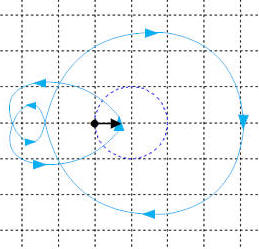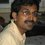# To really find a solution, Positively

During my research in Engineering, I came across this problem.

There is a complex valued function, with the domain as the imaginary axis.

$P(j\omega) = P_R(\omega^2)+j\omega P_I(\omega^2)$I need to find the value of $P(j\omega)$, at the sequence of points where it is real and draw appropriate conclusions.

There are two scenarios here. (1) Both $P_R(x)$ and $P_I(x)$ are polynomials in $x$. (2) Not case 1.

For case 1 : As we are interested in values of $P_R(\omega^2$) at those $\omega$, where $P_I(\omega^2)=0$, it is obvious that we are interested ONLY in positive and real solutions to $P_I(x)=0$.

My question to the community is this.

• Are there any methods to find the real roots (or better positive real roots) of a polynomial, without actually finding all the roots?*

If there is no simpler solution, than finding all the roots; then the procedure which I envision would become more computationally complex than a conventional technique that is more than 80 years old.

If case 1 is worth pursuing, then case 2 could be an interesting extension.Note by Janardhanan Sivaramakrishnan
5 years, 3 months ago

This discussion board is a place to discuss our Daily Challenges and the math and science related to those challenges. Explanations are more than just a solution — they should explain the steps and thinking strategies that you used to obtain the solution. Comments should further the discussion of math and science.

When posting on Brilliant:

• Use the emojis to react to an explanation, whether you're congratulating a job well done , or just really confused .
• Ask specific questions about the challenge or the steps in somebody's explanation. Well-posed questions can add a lot to the discussion, but posting "I don't understand!" doesn't help anyone.
• Try to contribute something new to the discussion, whether it is an extension, generalization or other idea related to the challenge.

MarkdownAppears as
*italics* or _italics_ italics
**bold** or __bold__ bold
- bulleted- list
• bulleted
• list
1. numbered2. list
1. numbered
2. list
Note: you must add a full line of space before and after lists for them to show up correctly
paragraph 1paragraph 2

paragraph 1

paragraph 2

[example link](https://brilliant.org)example link
> This is a quote
This is a quote
    # I indented these lines
# 4 spaces, and now they show
# up as a code block.

print "hello world"
# I indented these lines
# 4 spaces, and now they show
# up as a code block.

print "hello world"
MathAppears as
Remember to wrap math in $$ ... $$ or $ ... $ to ensure proper formatting.
2 \times 3 $2 \times 3$
2^{34} $2^{34}$
a_{i-1} $a_{i-1}$
\frac{2}{3} $\frac{2}{3}$
\sqrt{2} $\sqrt{2}$
\sum_{i=1}^3 $\sum_{i=1}^3$
\sin \theta $\sin \theta$
\boxed{123} $\boxed{123}$